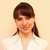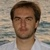# "Excel" category, page 12## How to count words in Excel

Excel doesn't provide any function or built-in tool for counting the number of words in a cell or range. Luckily, we can combine serval functions within a formula to get the desired result. In this tutorial, you will find a few useful formulas to count total or specific words in a cell or range. Continue reading## How to use LEN function in Excel to count characters in a cell

Are you looking for an Excel formula to count characters in a cell? If so, then you have certainly landed up on the right page. This short tutorial will teach you how you can use the LEN function to count characters in Excel, with or without spaces. Continue reading## How to split text string in Excel by comma, space, character or mask

Splitting text from one cell into several cells is the task all Excel users are dealing with once in a while. Today, we are going to take an in-depth look at how you can break strings into different elements using formulas and the Split Text feature. Continue reading## Excel Cumulative Sum - easy way to calculate running total

This short tutorial shows how a usual Excel Sum formula with a clever use of absolute and relative cell references can quickly calculate a running total in your worksheet. Continue reading## How to use AutoSum in Excel

Did you know that Excel SUM is the function that people read about most? No wonder they decided to add a special button to the Excel ribbon that inserts the SUM function automatically. So, if you wanted to know "What is AutoSum in Excel?" you already got the answer. Continue reading## Excel SUM formula to total a column, rows or only visible cells

The tutorial shows the most effective ways to use Excel's AutoSum feature and write your own Sum formulas to total a column, rows or selected range. You will also learn how to sum only visible cells, calculate running total and find out why your Sum formula is not working. Continue reading## How to create a histogram chart in Excel

While everyone knows how easy it is to create a chart in Excel, making a histogram usually raises a bunch of questions. In fact, in the recent versions of Excel 2010, Excel 2013, and Excel 2016, creating a histogram is a matter of minutes and can be done in a variety of ways. Continue reading## Excel SWITCH function - the compact form of nested IF statement

This article introduces you to the Excel SWITCH function, describes its syntax and provides a couple of use cases to illustrate how you can simplify writing nested IFs in Excel. Continue reading## How to get a list of unique and distinct values in Excel

If you want to get a list of unique values in a column, this tutorial offers a number of formulas and will teach you how to tweak them for different datasets. You will also learn how to quickly get a distinct list using Excel's Advanced Filter, and how to extract unique rows with Duplicate Remover. Continue reading## Distinct and unique values in Excel: how to find, filter, select and highlight

If you want to quickly find, filter and highlight unique or distinct values in your Excel worksheets, this tutorial will show you fast and efficient ways. Continue reading## How to count unique values in Excel

In this tutorial, you will learn how to count unique values in Excel with formulas, and how to get an automatic count of distinct values in a pivot table. We will also discuss a number of formula examples for counting unique names, texts, numbers, cased-sensitive unique values, and more. Continue reading## Excel COUNT and COUNTA functions to count cells

This short tutorial explains the basics of the Excel COUNT and COUNTA functions and shows a few examples of using a count formula in Excel. You will also learn how to use the COUNTIF and COUNTIFS functions to count cells that meet one or more criteria. Continue reading## How to remove duplicates in Excel (duplicate rows, values and partial matches)

The tutorial explains how to remove duplicates in Excel 2010, Excel 2013, and Excel 2016. You will learn a few different techniques to find and delete duplicate values with or without first occurrences, remove duplicate rows, detect absolute duplicates and partial matches. Continue reading## How to highlight duplicate cells and rows in Excel

Today, we are going to have a close look at how to show duplicates in Excel. You will learn how to shade duplicate cells, entire rows, or consecutive dupes using conditional formatting. Also, you will see how to highlight duplicates with different colors using a specialized tool. Continue reading## How to find duplicates in Excel: identify, highlight, count, filter

The tutorial explains how to search for duplicates in Excel. You will learn a few formulas to identify duplicate values or find duplicate rows with or without first occurrences. You will also learn how to count instances of each duplicate record individually and find the total number of dupes in a column, how to filter duplicates, and more. Continue reading## How to compare two Excel files for differences

Learn a variety of methods to compare Excel files and identify differences between them. See how to open two Excel windows side by side, how to use Excel formulas to create a difference report, highlight differences with conditional formatting, and more. Continue reading## How to lock and unlock cells in Excel

Learn a few useful techniques to lock a cell or a few cells in Excel to protect them from deleting, overwriting or editing. The tutorial also shows how to unlock individual cells on a protected sheet by a password, or allow specific users to edit those cells without password. And finally, you will learn how to detect and highlight locked and unlocked cells in Excel. Continue reading## How to protect and unprotect Excel sheet with or without password

The tutorial shows the detailed steps to protect Excel sheet with or without passwords. It also explains how to unlock Excel spreadsheet without password if you forgot or misplaced it. Continue reading## Excel formulas not working, not updating, not calculating: fixes & solutions

This tutorial explains the most common mistakes when making formulas in Excel, and how to fix a formula that is not calculating or not updating automatically. Continue reading## How to edit, evaluate and debug formulas in Excel

In this tutorial, you will find a few quick and efficient ways to check and debug formulas in Excel. See how to use the F9 key to evaluate formula parts, how to highlight cells that reference or are referenced by a given formula, how to determine mismatched or misplaced parentheses, and more. Continue reading## Formula bar in Excel: how to show, hide, expand or collapse

In this short tutorial, you will learn what the Excel formula bar is, how to restore a missing formula bar in different versions of Excel, and how to expand the formula bar so that a long formula can fit into it entirely. Continue reading## How to copy formula in Excel: down a column, without changing references, etc.

In this tutorial, you will learn a few different ways of copying formulas in Excel: how to copy formula down a column, to all of the selected cells, copy a formula exactly without changing cell references or formatting, and more. Continue reading## How to hide and lock formulas in Excel

This tutorial shows how to hide formulas in Excel so they do not show up in the formula bar. Also, you will learn how to quickly lock a selected formula or all formulas in a worksheet to protect them from being deleted or overwritten by other users. Continue reading## How to show formulas in Excel

In this short tutorial, you will learn an easy way to show formulas in Excel 2016, 2013, 2010 and older versions. Also, you will learn how to print formulas and why sometimes Excel shows a formula, not result, in a cell. Continue reading## How to make formulas in Excel

The tutorial explains how to write formulas in Excel, beginning with very simple ones. You will learn how to create a formula in Excel using constants, cell references and defined names. Also, you will see how to make formulas using … Continue reading## Microsoft Excel formulas with examples

Can Microsoft Excel formulas be easy to learn? Yep! This tutorial explains the very basics of Excel formulas for beginners, with detailed steps on how to write and use them. It also provides a number of advanced formula examples for experienced users. You will be amazed how simple creating formulas in Excel actually is. Continue reading## Circular reference in Excel - how to check, find, enable, or remove

This short tutorial explains the basics of Excel circular reference and why you should beware of using them. You will also learn how to check, find and remove circular references in Excel worksheets, and if none of the above is an option, how to enable and use circular formulas. Continue reading## 3-D reference in Excel: reference the same cell or range in multiple worksheets

This short tutorial explains the essence of Excel 3-D reference and shows how you can calculate multiple worksheets with a single 3-D formula. Continue reading## How to create external reference in Excel to refer to another sheet or workbook

This short tutorial explains the basics of an external reference in Excel, and shows how to reference another sheet and workbook in your formulas. Continue reading## Why use dollar sign (\$) in Excel formulas - absolute and relative cell references

When writing an Excel formula, \$ sign in cell references confuses many users. But the explanation is very simple. The dollar sign in an Excel cell reference serves just one purpose - it tells Excel whether to change or not to change the reference when the formula is copied to other cells. And this short tutorial provides full details about this great feature. Continue reading